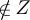Algebraic General Topology. Vol 1: Paperback / E-book || Axiomatic Theory of Formulas: Paperback / E-book

As an Amazon Associate I earn from qualifying purchases.

Free stars
by Victor Porton
Shay Agnon 32-29
Email: porton@narod.ru
Web: http://www.mathematics21.org
August 26, 2012
Abstract
Free stars are certain subsets of a poset, rst dened by me in . Free stars are closely
related with lters, namely free stars on a boolean lattice bijectively correspond to lters on
this lattice. This article introduces consideration of free stars. Some questions are raised.
Free stars are useful in my further (yet unpublished) research (in general topology).
Keywords: lters, ltrators, posets, lattices
A.M.S. subject classiﬁcation: 06A06, 06B99
1 Notation
Partial order is denoted as .
Meets and joins are denoted as , ,
d
,
F
.
I don’t use in this article the concept of ﬁlter objects , but instead just deﬁne the order on
a lattice of ﬁlters reverse to set-theoretic inclusion: A B A B.
2 Deﬁnition
I recall the deﬁnition from :
Deﬁnition 1. Let A be a poset. Free stars on A are such S P A that the least element (if it
exists) is not in S and for every X , Y A
Z A: (Z X Z Y Z S) X S Y S.
Proposition 2 . S PA where A is a poset is a free star iﬀ all of the following:
1. The least element (if it exists) is not in S.
2. Z A: (Z X Z Y Z S) X S Y S f or every X , Y A.
3. S is an upper set.
Proof. See .
Proposition 3 . Let A be a join-semilat tice. S P A is a free star iﬀ all of the following:
1. The least element (if it exists) is not in S.
2. X Y S X S Y S for every X , Y A.
3. S is an upper set.
Proof. See .
Proposition 4. Let A be a join-semilattice. S PA is a free star iﬀ the least element (if it exists)
is not in S and for every X , Y A
X Y S X S Y S.
1Proof. See .
See  for deﬁnition and properties of full stars and core sta rs. There it is also described a
bijection betwee n free stars on a boolean lattice and lters on that lattice (theorems 43, 44, and
45 in ).
3 Orde r of free stars
The set of free stars is ordered by the pa rtial order deﬁned by the formula S T S T .
Obvious 5. The minimal free sta r is .
Proposition 6 . The maximal free star is A \ {0} (or A if 0 doesn’t exist).
Proof. Let’s denote our star S (that is S = A \ {0} or S = A depe ndently on existence of 0).
It is enough to prove that S is a free star.
That the least element is not in S is obvious. That S is an upper set is obvious.
The only thing remained to prove is
Z A: (Z X Z Y Z S) X S Y S.
If X or Y are non-least, it is obvious. Then only case r e mained to consider is X = Y = 0. In this
case our formula takes the form:
Z A: Z S X S Y S what is true because Z A: Z S is false (0
Z).
Proposition 7 . If S is a set of free stars on a join-semilattice, then
S
S is a free star.
Proof. Let S is a set of f ree stars on a join-semilatt ic e .
Obviously
S
S does not co ntain the least element (if it exists) and
S
S is an upper set.
It is remained to prove that X Y
S
S X
S
S Y
S
S.
Let X Y
S
S. Then there e xists T S such that X Y T . Hence X T Y T and thus
X
S
S Y
S
S.
Conjecture 8. This proposition cannot be strengthened for arbitrary posets instead of join-
semilattices.
Next two corollaries from the above proposition:
Corollary 9.
F
S =
S
S if S is a set of free stars on a join-semilattice.
Corollary 10. The set of free stars on a join-semilattice is a complete lattice.
4 Informal open problems
For which kinds of posets the corresponding poset of free stars is :
1. a distributive lattice?
2. a co-brouwe rian lattice?
3. an atomic poset?
4. an atomistic poset?
5. a separable poset? (see  for a deﬁnition)
6. an atomically separable poset? (see  for a deﬁnition)
Answers to these questions for the poset of all subsets of a ﬁxed set are obv iously all true, due the
mentioned above bijective corres pondence with the set of ﬁlte rs on that poset and results in .
Can these results be signiﬁcantly strengthened?
Bib liography
 Victor Porton. Filters on posets and generalizations. International Journal of Pure and Applied Mathe-
matics, 74(1):55–119, 2012.
2 Section